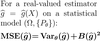Level 27 Level 29
Level 28

## Ignore words

Check the boxes below to ignore/unignore words, then click save at the bottom. Ignored words will never appear in any learning session.

Ignore?
Statistical model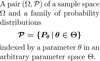Estimator (of a parameter)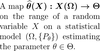Estimand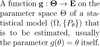Estimator (function)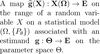Sample estimateSet of sample estimates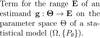Likelihood function (discrete)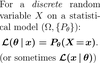Likelihood function (continuous)Log-likelihood function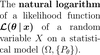Maximum likelihood estimator (MLE)Maximum likelihood equation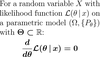Expected value (of an estimator)Bias (of an estimator)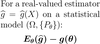Mean squared error (MSE)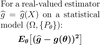Variance (of an estimator)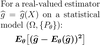Root-mean-square error (RMSE)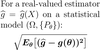Standard error (of an estimator)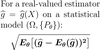"MSE vs variance and bias"